Скачать презентацию Lesson 2 4 Logical Sequencing Conditional Statements

• Количество слайдов: 16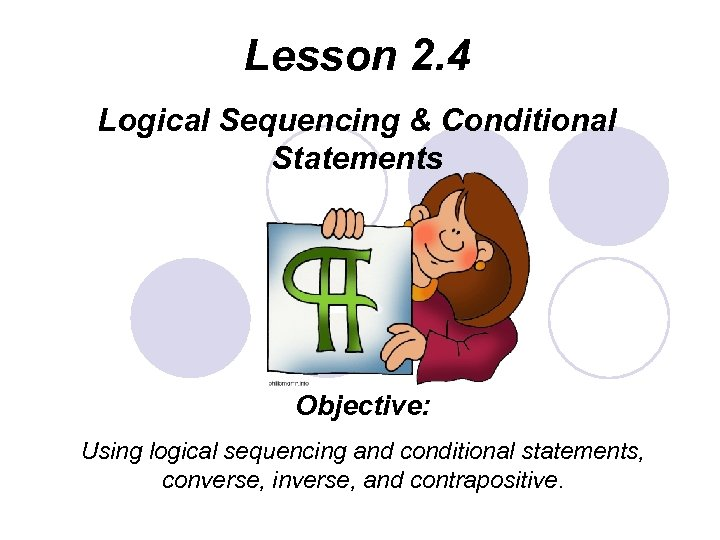Lesson 2. 4 Logical Sequencing & Conditional Statements Objective: Using logical sequencing and conditional statements, converse, inverse, and contrapositive.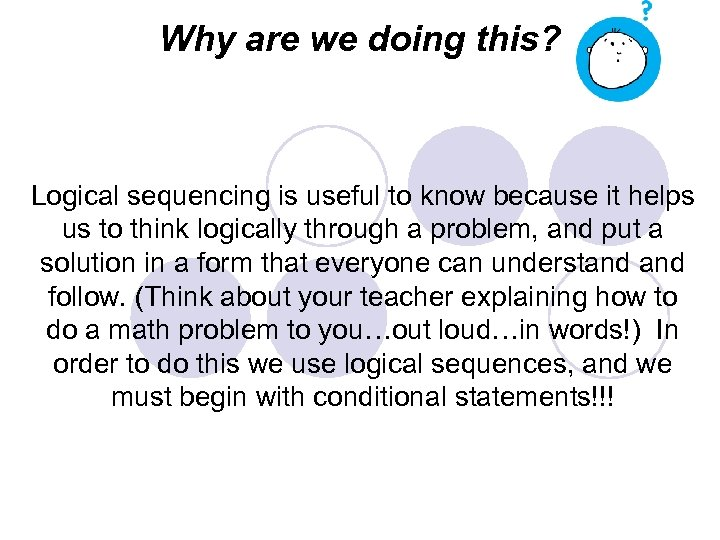Why are we doing this? Logical sequencing is useful to know because it helps us to think logically through a problem, and put a solution in a form that everyone can understand follow. (Think about your teacher explaining how to do a math problem to you…out loud…in words!) In order to do this we use logical sequences, and we must begin with conditional statements!!!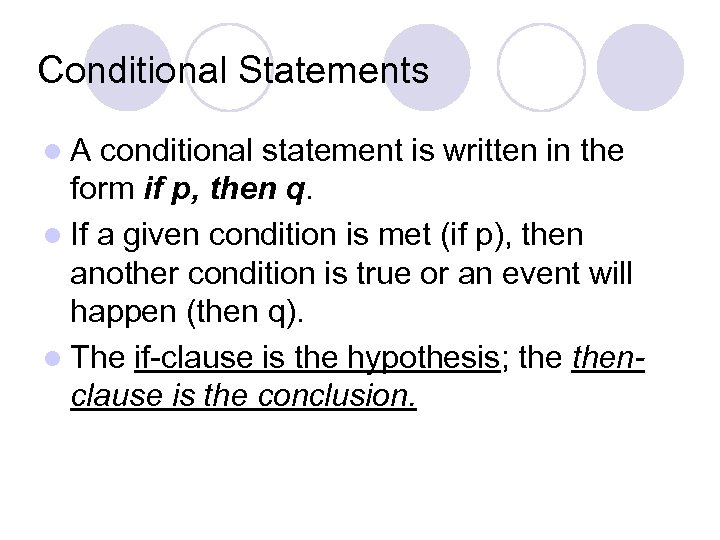Conditional Statements l. A conditional statement is written in the form if p, then q. l If a given condition is met (if p), then another condition is true or an event will happen (then q). l The if-clause is the hypothesis; thenclause is the conclusion.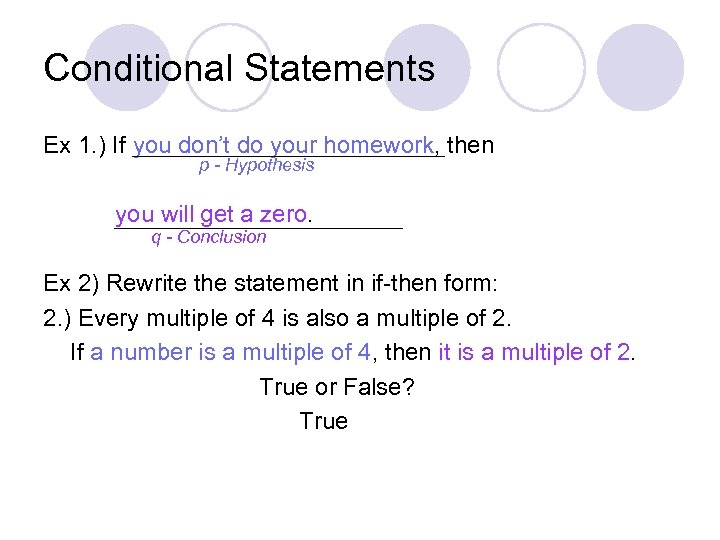Conditional Statements Ex 1. ) If you don’t do your homework, then p - Hypothesis you will get a zero. q - Conclusion Ex 2) Rewrite the statement in if-then form: 2. ) Every multiple of 4 is also a multiple of 2. If a number is a multiple of 4, then it is a multiple of 2. True or False? True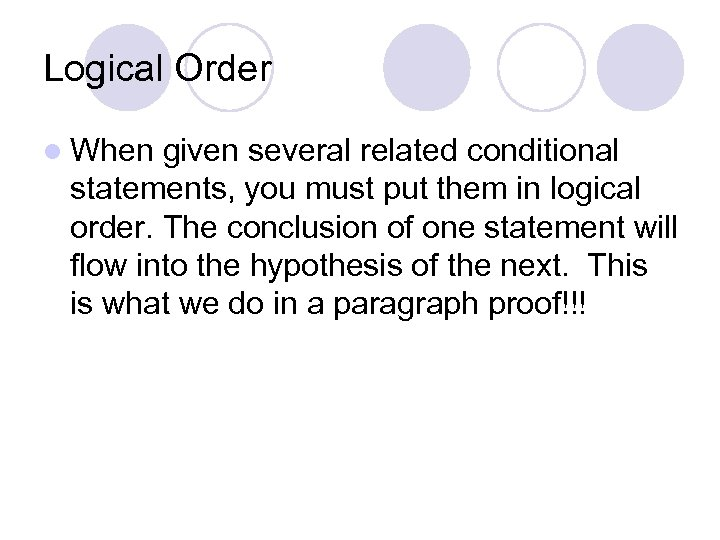Logical Order l When given several related conditional statements, you must put them in logical order. The conclusion of one statement will flow into the hypothesis of the next. This is what we do in a paragraph proof!!!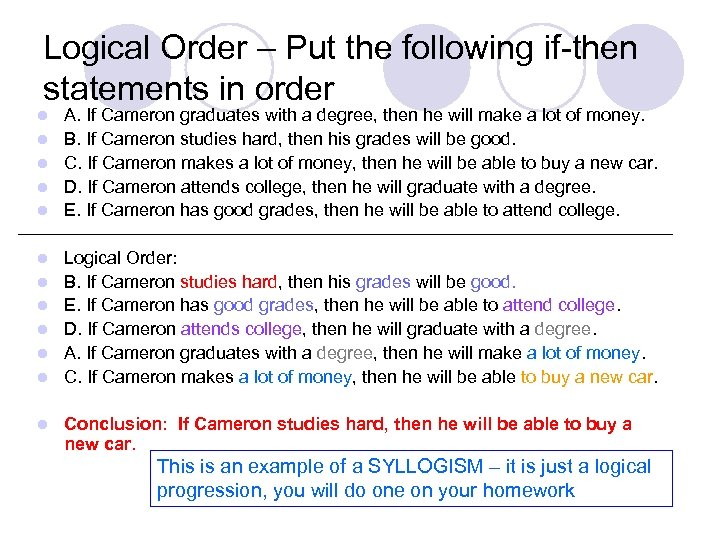Logical Order – Put the following if-then statements in order l l l A. If Cameron graduates with a degree, then he will make a lot of money. B. If Cameron studies hard, then his grades will be good. C. If Cameron makes a lot of money, then he will be able to buy a new car. D. If Cameron attends college, then he will graduate with a degree. E. If Cameron has good grades, then he will be able to attend college. Logical Order: B. If Cameron studies hard, then his grades will be good. E. If Cameron has good grades, then he will be able to attend college. D. If Cameron attends college, then he will graduate with a degree. A. If Cameron graduates with a degree, then he will make a lot of money. C. If Cameron makes a lot of money, then he will be able to buy a new car. Conclusion: If Cameron studies hard, then he will be able to buy a new car. This is an example of a SYLLOGISM – it is just a logical progression, you will do one on your homework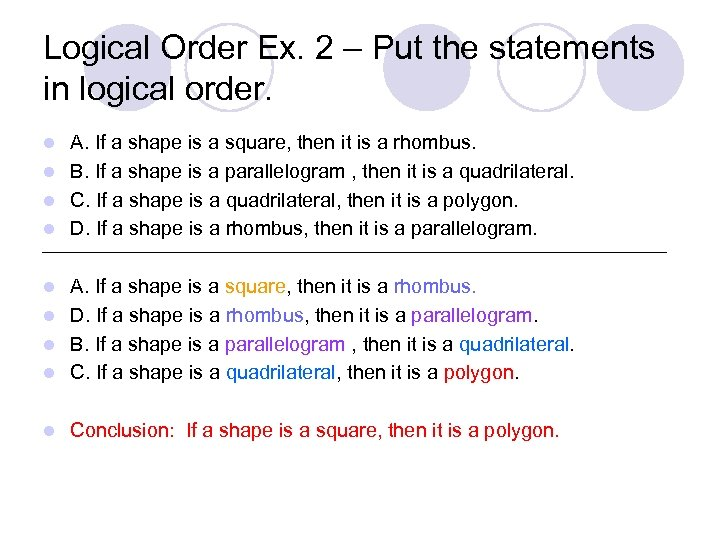Logical Order Ex. 2 – Put the statements in logical order. A. If a shape is a square, then it is a rhombus. l B. If a shape is a parallelogram , then it is a quadrilateral. l C. If a shape is a quadrilateral, then it is a polygon. l D. If a shape is a rhombus, then it is a parallelogram. l A. If a shape is a square, then it is a rhombus. l D. If a shape is a rhombus, then it is a parallelogram. l B. If a shape is a parallelogram , then it is a quadrilateral. l C. If a shape is a quadrilateral, then it is a polygon. l l Conclusion: If a shape is a square, then it is a polygon.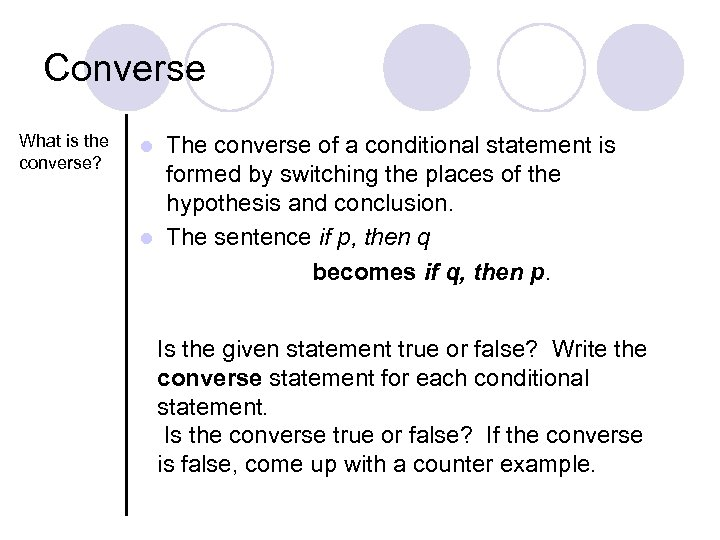Converse What is the converse? The converse of a conditional statement is formed by switching the places of the hypothesis and conclusion. l The sentence if p, then q becomes if q, then p. l Is the given statement true or false? Write the converse statement for each conditional statement. Is the converse true or false? If the converse is false, come up with a counter example.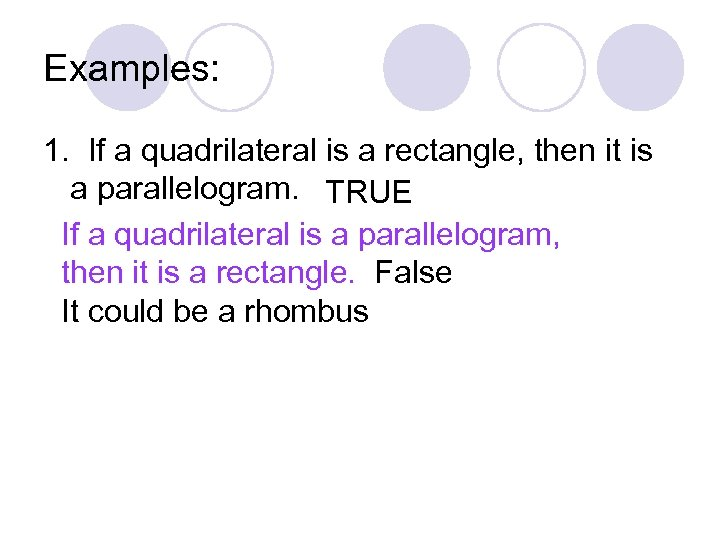Examples: 1. If a quadrilateral is a rectangle, then it is a parallelogram. TRUE If a quadrilateral is a parallelogram, then it is a rectangle. False It could be a rhombus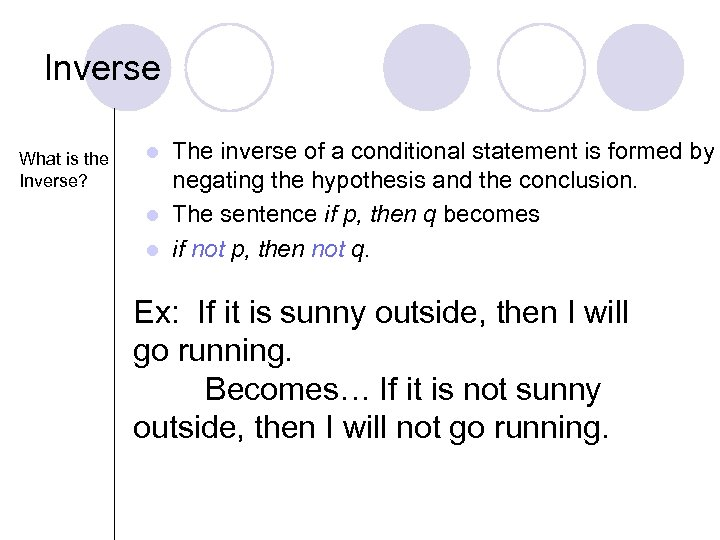Inverse What is the Inverse? The inverse of a conditional statement is formed by negating the hypothesis and the conclusion. l The sentence if p, then q becomes l if not p, then not q. l Ex: If it is sunny outside, then I will go running. Becomes… If it is not sunny outside, then I will not go running.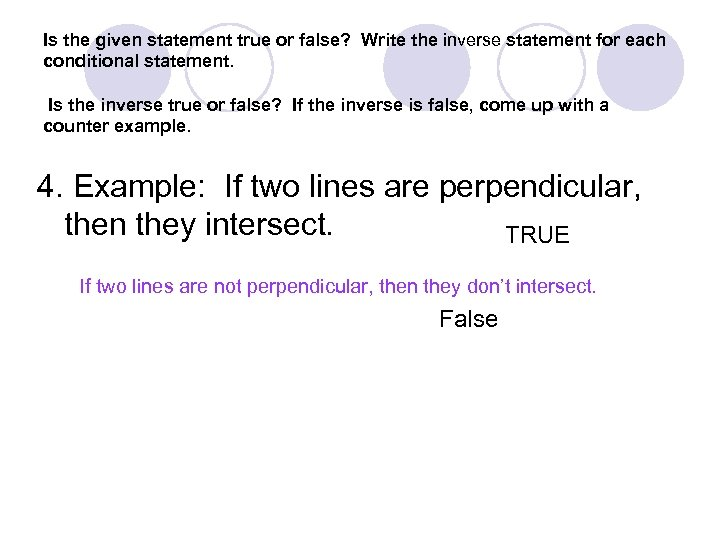Is the given statement true or false? Write the inverse statement for each conditional statement. Is the inverse true or false? If the inverse is false, come up with a counter example. 4. Example: If two lines are perpendicular, then they intersect. TRUE If two lines are not perpendicular, then they don’t intersect. False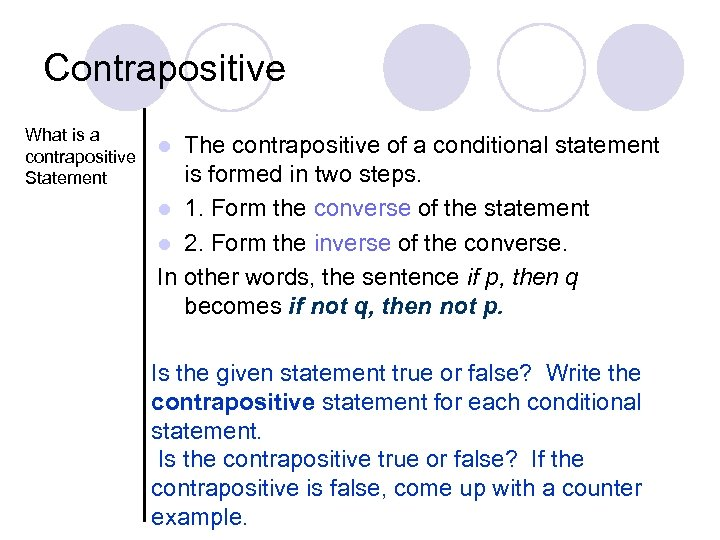Contrapositive What is a contrapositive Statement The contrapositive of a conditional statement is formed in two steps. l 1. Form the converse of the statement l 2. Form the inverse of the converse. In other words, the sentence if p, then q becomes if not q, then not p. l Is the given statement true or false? Write the contrapositive statement for each conditional statement. Is the contrapositive true or false? If the contrapositive is false, come up with a counter example.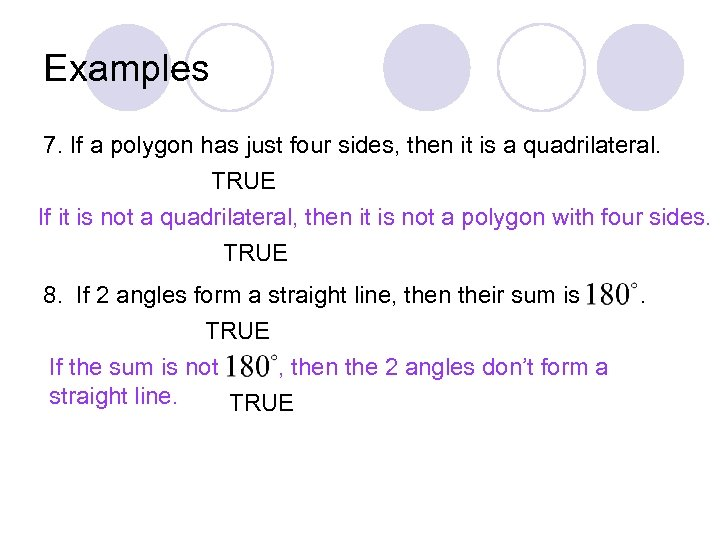Examples 7. If a polygon has just four sides, then it is a quadrilateral. TRUE If it is not a quadrilateral, then it is not a polygon with four sides. TRUE 8. If 2 angles form a straight line, then their sum is TRUE If the sum is not , then the 2 angles don’t form a straight line. TRUE .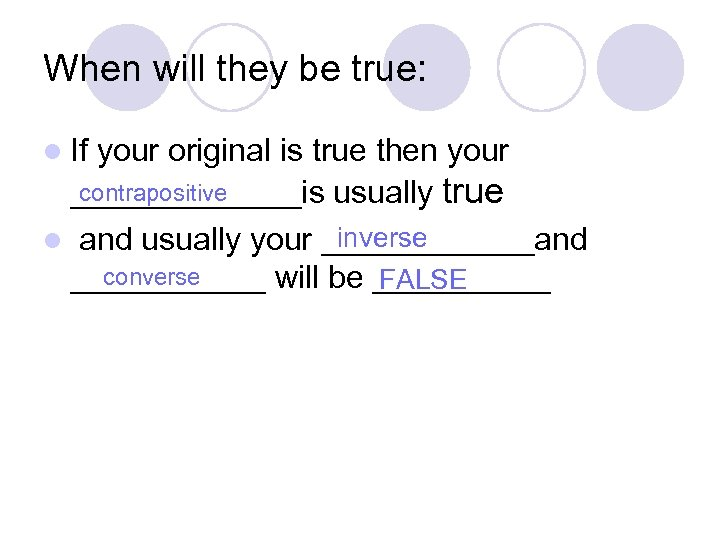When will they be true: l If your original is true then your contrapositive _______is usually true inverse l and usually your ______and converse ______ will be _____ FALSE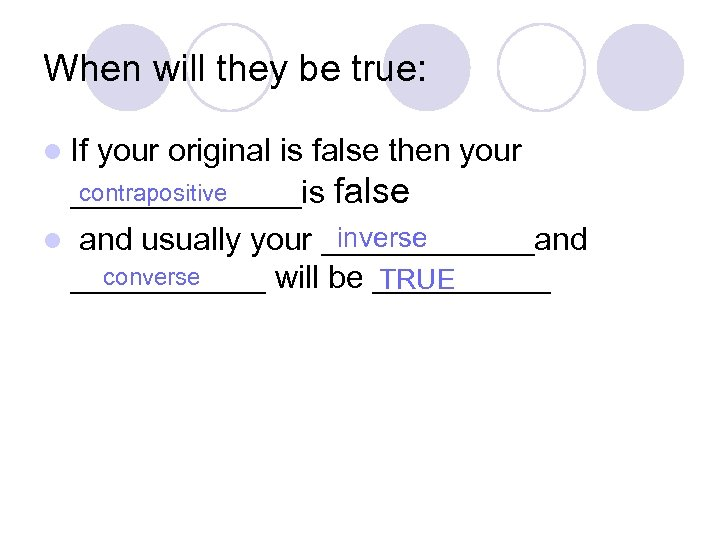When will they be true: l If your original is false then your contrapositive _______is false inverse l and usually your ______and converse ______ will be _____ TRUE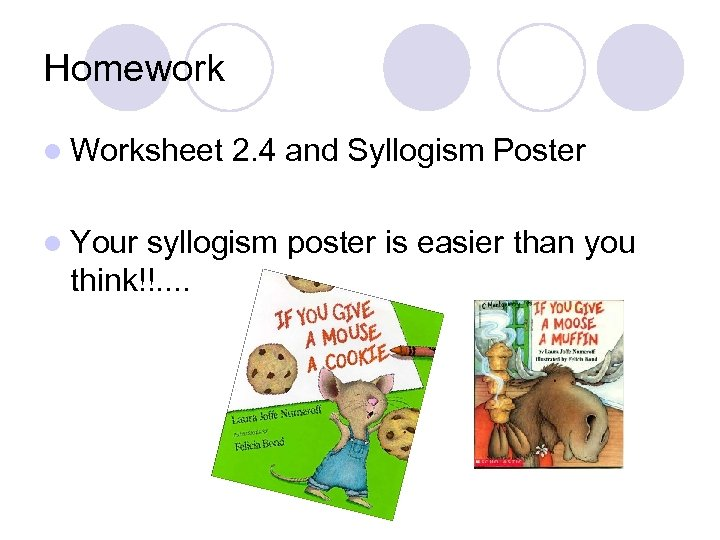Homework l Worksheet l Your 2. 4 and Syllogism Poster syllogism poster is easier than you think!!. .